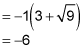##### Differential Equations Workbook For Dummies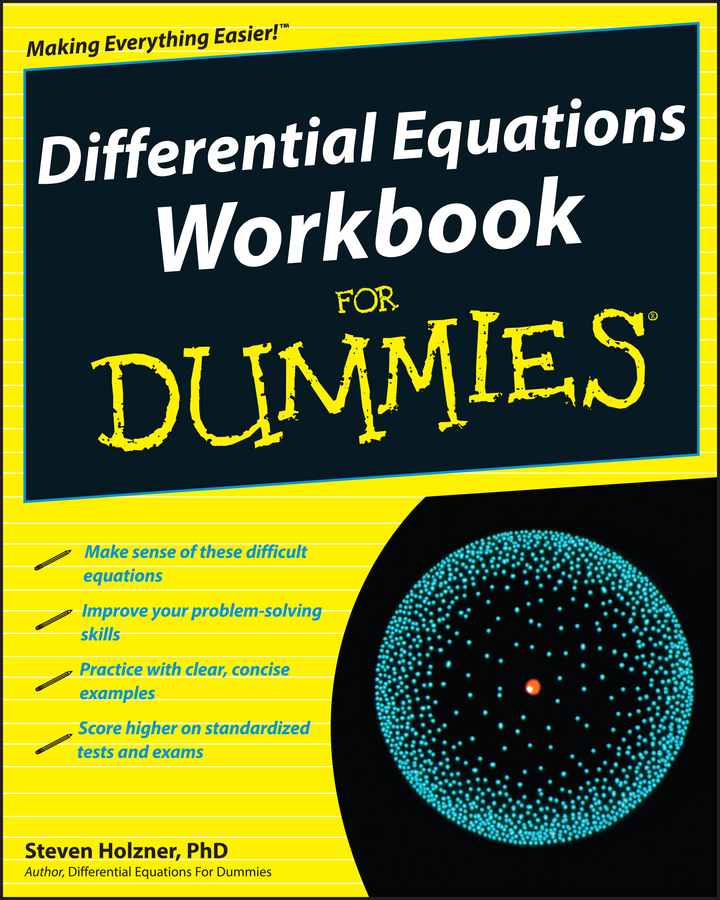When simply plugging the arrow number into a limit expression doesn't work, you can solve a limit problem using a range of algebraic techniques. These can include factoring, cancelling and conjugate multiplication.

Of course, before you try any algebra, your first step should always be to plug the arrow-number into the limit expression. If the function is continuous at the arrow-number (which it usually will be) and if plugging in results in an ordinary number, then that's the answer. You're done. For example, to evaluate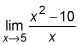just plug in the arrow-number. You get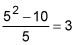That's all there is to it. Don't forget to plug in!

When plugging in fails because it gives you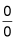you've got a nontrivial limit problem and a bit of work to do. You have to convert the fraction into some expression where plugging in does work. Here are some algebraic methods you can try:

• FOILing

• Factoring

• Finding the least common denominator

• Canceling

• Simplification

• Conjugate multiplication

Some of these methods are illustrated in the following examples.

## Practice questions

1. Solve the following limit: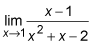2. Solve the following limit: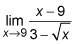To obtain the answer, you need to factor, cancel, and plug in.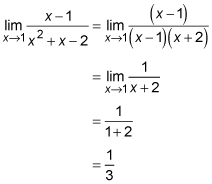This one is a bit more involved.

You start by multiplying the numerator and denominator by the conjugate of the denominator,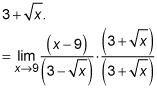Now multiply out the part of the fraction containing the conjugate pair (the denominator in this problem).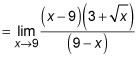Cancel.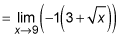Remember that any fraction of the form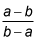always equals –1.

Now plug in.